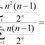# Interesting Sum

$\large { \left( \tan { x } \right) }^{ 2 }={ \left( \sin { x } \right) }^{ 2 }{ +\left( \sin { x } \right) }^{ 4 }+{ \left( \sin { x } \right) }^{ 6 }+{ \left( \sin { x } \right) }^{ 8 }+\cdots$

Prove the trigonometric identity above.Note by Joel Yip
5 years, 5 months ago

This discussion board is a place to discuss our Daily Challenges and the math and science related to those challenges. Explanations are more than just a solution — they should explain the steps and thinking strategies that you used to obtain the solution. Comments should further the discussion of math and science.

When posting on Brilliant:

• Use the emojis to react to an explanation, whether you're congratulating a job well done , or just really confused .
• Ask specific questions about the challenge or the steps in somebody's explanation. Well-posed questions can add a lot to the discussion, but posting "I don't understand!" doesn't help anyone.
• Try to contribute something new to the discussion, whether it is an extension, generalization or other idea related to the challenge.

MarkdownAppears as
*italics* or _italics_ italics
**bold** or __bold__ bold
- bulleted- list
• bulleted
• list
1. numbered2. list
1. numbered
2. list
Note: you must add a full line of space before and after lists for them to show up correctly
paragraph 1paragraph 2

paragraph 1

paragraph 2

[example link](https://brilliant.org)example link
> This is a quote
This is a quote
    # I indented these lines
# 4 spaces, and now they show
# up as a code block.

print "hello world"
# I indented these lines
# 4 spaces, and now they show
# up as a code block.

print "hello world"
MathAppears as
Remember to wrap math in $$ ... $$ or $ ... $ to ensure proper formatting.
2 \times 3 $2 \times 3$
2^{34} $2^{34}$
a_{i-1} $a_{i-1}$
\frac{2}{3} $\frac{2}{3}$
\sqrt{2} $\sqrt{2}$
\sum_{i=1}^3 $\sum_{i=1}^3$
\sin \theta $\sin \theta$
\boxed{123} $\boxed{123}$

Sort by:

$\boxed{\boxed{\boxed{\boxed{\boxed{\boxed{\boxed{\boxed{\boxed{\boxed{\boxed{\boxed{\boxed{\boxed{\boxed{\boxed{\boxed{\boxed{\boxed{\boxed{\boxed{\boxed{\boxed{\boxed{\boxed{\boxed{\boxed{\boxed{\boxed{\boxed{\boxed{\boxed{\boxed{\boxed{\boxed{\boxed{\boxed{\boxed{\boxed{\boxed{\boxed{\boxed{\boxed{\boxed{\boxed{\boxed{\boxed{\boxed{\boxed{\boxed{\boxed{\boxed{\boxed{\boxed{\boxed{\boxed{\boxed{\boxed{\boxed{\boxed{\boxed{\boxed{\boxed{\boxed{\boxed{\boxed{\boxed{\boxed{\boxed{\boxed{\boxed{\boxed{\boxed{\boxed{\boxed{\boxed{\boxed{\boxed{S=\dfrac{\sin^2 x}{1-\sin^2 x} = \dfrac{\sin^2x}{\cos^2 x} = \boxed{\tan^2x} }}}}}}}}}}}}}}}}}}}}}}}}}}}}}}}}}}}}}}}}}}}}}}}}}}}}}}}}}}}}}}}}}}}}}}}}}}}}}}$

- 5 years, 5 months ago

wow it looks like a pyramid. How long did you take to type and count so many boxes :)?

- 5 years, 5 months ago

- 5 years, 5 months ago

100% vella! Just like @Mehul Arora

- 5 years, 2 months ago

Haha, I actually copy pasted the latex from some other note :P

It is related to Andrew's recent problem :3

- 5 years, 2 months ago

The sum of an infinite GP can only be calculated when $-1. However $\sin^2x$ can be equal to 1. Can you justify?

- 5 years, 2 months ago

It should be specified that $|\sin x|<1$

- 5 years, 2 months ago

Well it is already in the range!

- 5 years, 2 months ago

Interesting!

- 5 years, 4 months ago